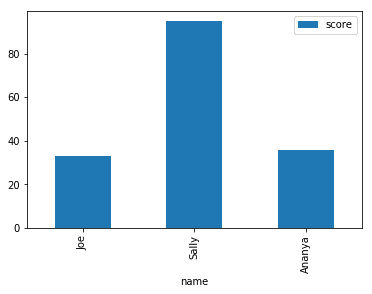December 20, 2017

# Adding Axis Labels to Plots With pandas

Pandas plotting methods provide an easy way to plot pandas objects. Often though, you’d like to add axis labels, which involves understanding the intricacies of Matplotlib syntax. Thankfully, there’s a way to do this entirely using pandas. Let’s start by importing the required libraries:

``````import pandas as pd
import numpy as np
import matplotlib.pyplot as plt``````

Next, we’ll create a test dataframe, with a column of names and corresponding test scores:

``````df = pd.DataFrame({
"name": ["Joe", "Sally", "Ananya"],
"score": np.random.randint(0,100,size=3)})
df``````
name score
0 Joe 42
1 Sally 37
2 Ananya 98

Plotting this dataframe is simple with the pandas methods. We can just use the DataFrame.plot.bar() method to produce a bar plot in two lines.

``````df.plot.bar()
plt.show()``````This gives us instant results, but it’s not easy to interpret this plot, because we can’t see which scores belong to which name. If you look closely, you might notice the currently x-axis labels are `0`, `1`, and `2`. These actually correspond with the dataframe index. By setting the index of the dataframe to our names using the `set_index()` method, we can easily produce axis labels and improve our plot. We’ll use `drop=True` which will remove the column, and `inplace=True` instead of having to assign the variable back to itself or to a new variable name.

``````df.set_index("name",drop=True,inplace=True)
df``````
score
name
Joe 33
Sally 95
Ananya 36

Now, let’s plot again and see our results:

``````df.plot.bar()
plt.show()``````Instantly, our plot is better looking, and clearly communicates the data!

If you’re interested in learning more about data visualization in Python, you might like to check ourData Visualization with Matplotlib course.